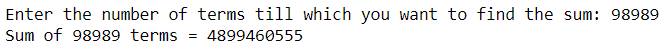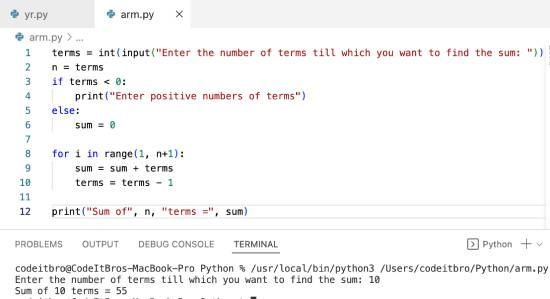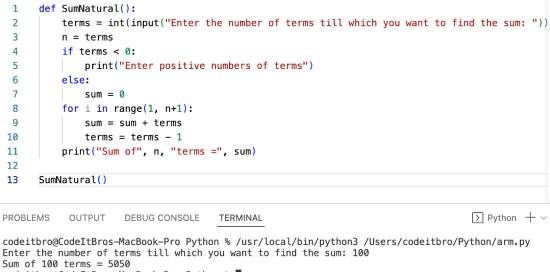# Python 3 Program to Find the Sum of Natural Numbers

Here, I will share a simple Python 3 program to find the sum of natural numbers. Before moving ahead, you should understand the basic Python programming language concepts such as variables, data types, and if-else loop statements.

## Python program to find the sum of n natural numbers using while loop

In this Python program, we will first take an input to store the number of terms till which we have to calculate the sum. Next, we will check if the user input is positive or negative. If the input is negative, then the user will see the message “Enter positive number of terms,” and if the input is positive, Python will start executing the while loop.

The while loop calculates the sum of terms until the term is less than zero. After all the iterations of the while loop are complete, it will display the sum of n natural numbers.

```terms = int(input("Enter the number of terms till which you want to find the sum: "))
n = terms
if terms < 0:
print("Enter positive numbers of terms")
else:
sum = 0
while (terms > 0):
sum = sum + terms
terms = terms - 1

print("Sum of", n, "terms =", sum)```

Try it yourself:

Output:

Enter the number of terms till which you want to find the sum: 98989

Sum of 98989 terms = 4899460555## Python program to find the sum of n natural numbers using for loop

Code:

```terms = int(input("Enter the number of terms till which you want to find the sum: "))
n = terms
if terms < 0:
print("Enter positive numbers of terms")
else:
sum = 0

for i in range(1, n+1):
sum = sum + terms
terms = terms - 1

print("Sum of", n, "terms =", sum)```

Output:## Python program to find the sum of n numbers using function

Code:

```def SumNatural():
terms = int(input("Enter the number of terms till which you want to find the sum: "))
n = terms
if terms < 0:
print("Enter positive numbers of terms")
else:
sum = 0
for i in range(1, n+1):
sum = sum + terms
terms = terms - 1
print("Sum of", n, "terms =", sum)

SumNatural()```

Output:Scroll to Top